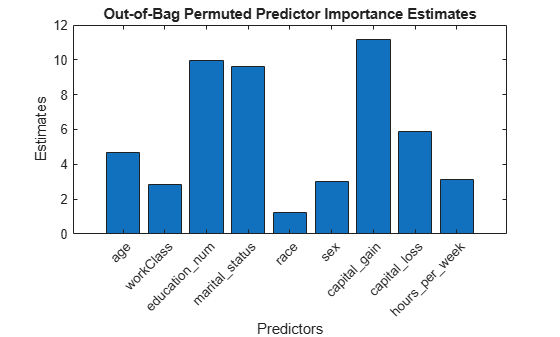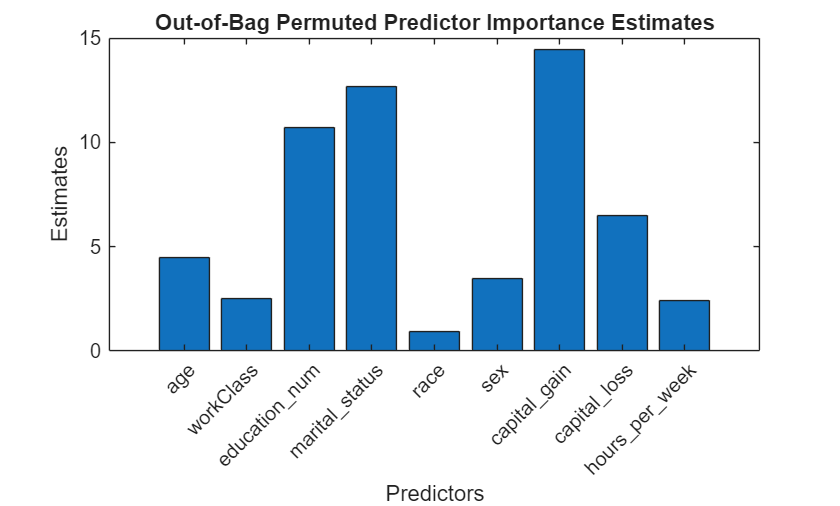# oobPermutedPredictorImportance

Predictor importance estimates by permutation of out-of-bag predictor observations for random forest of classification trees

## Syntax

``Imp = oobPermutedPredictorImportance(Mdl)``
``Imp = oobPermutedPredictorImportance(Mdl,Name,Value)``

## Description

example

````Imp = oobPermutedPredictorImportance(Mdl)` returns a vector of out-of-bag, predictor importance estimates by permutation using the random forest of classification trees `Mdl`. `Mdl` must be a `ClassificationBaggedEnsemble` model object.```

example

````Imp = oobPermutedPredictorImportance(Mdl,Name,Value)` uses additional options specified by one or more `Name,Value` pair arguments. For example, you can speed up computation using parallel computing or indicate which trees to use in the predictor importance estimation.```

## Input Arguments

expand all

Random forest of classification trees, specified as a `ClassificationBaggedEnsemble` model object created by `fitcensemble`.

### Name-Value Arguments

Specify optional pairs of arguments as `Name1=Value1,...,NameN=ValueN`, where `Name` is the argument name and `Value` is the corresponding value. Name-value arguments must appear after other arguments, but the order of the pairs does not matter.

Before R2021a, use commas to separate each name and value, and enclose `Name` in quotes.

Indices of learners to use in predictor importance estimation, specified as the comma-separated pair consisting of `'Learners'` and a numeric vector of positive integers. Values must be at most `Mdl.NumTrained`. When `oobPermutedPredictorImportance` estimates the predictor importance, it includes the learners in `Mdl.Trained(learners)` only, where `learners` is the value of `'Learners'`.

Example: `'Learners',[1:2:Mdl.NumTrained]`

Parallel computing options, specified as the comma-separated pair consisting of `'Options'` and a structure array returned by `statset`. `'Options'` requires a Parallel Computing Toolbox™ license.

`oobPermutedPredictorImportance` uses the `'UseParallel'` field only. `statset('UseParallel',true)` invokes a pool of workers.

Example: `'Options',statset('UseParallel',true)`

## Output Arguments

expand all

Out-of-bag, predictor importance estimates by permutation, returned as a 1-by-p numeric vector. p is the number of predictor variables in the training data (`size(Mdl.X,2)`). `Imp(j)` is the predictor importance of the predictor `Mdl.PredictorNames(j)`.

## Examples

expand all

Load the `census1994` data set. Consider a model that predicts a person's salary category given their age, working class, education level, martial status, race, sex, capital gain and loss, and number of working hours per week.

```load census1994 X = adultdata(:,{'age','workClass','education_num','marital_status','race',... 'sex','capital_gain','capital_loss','hours_per_week','salary'});```

You can train a random forest of 50 classification trees using the entire data set.

```Mdl = fitcensemble(X,'salary','Method','Bag','NumLearningCycles',50); ```

`fitcensemble` uses a default template tree object `templateTree()` as a weak learner when `'Method'` is `'Bag'`. In this example, for reproducibility, specify `'Reproducible',true` when you create a tree template object, and then use the object as a weak learner.

```rng('default') % For reproducibility t = templateTree('Reproducible',true); % For reproducibiliy of random predictor selections Mdl = fitcensemble(X,'salary','Method','Bag','NumLearningCycles',50,'Learners',t);```

`Mdl` is a `ClassificationBaggedEnsemble` model.

Estimate predictor importance measures by permuting out-of-bag observations. Compare the estimates using a bar graph.

```imp = oobPermutedPredictorImportance(Mdl); figure; bar(imp); title('Out-of-Bag Permuted Predictor Importance Estimates'); ylabel('Estimates'); xlabel('Predictors'); h = gca; h.XTickLabel = Mdl.PredictorNames; h.XTickLabelRotation = 45; h.TickLabelInterpreter = 'none';````imp` is a 1-by-9 vector of predictor importance estimates. Larger values indicate predictors that have a greater influence on predictions. In this case, `marital_status` is the most important predictor, followed by `capital_gain`.

Load the `census1994` data set. Consider a model that predicts a person's salary category given their age, working class, education level, martial status, race, sex, capital gain and loss, and number of working hours per week.

```load census1994 X = adultdata(:,{'age','workClass','education_num','marital_status','race', ... 'sex','capital_gain','capital_loss','hours_per_week','salary'});```

Display the number of categories represented in the categorical variables using `summary`.

`summary(X)`
```Variables: age: 32561×1 double Values: Min 17 Median 37 Max 90 workClass: 32561×1 categorical Values: Federal-gov 960 Local-gov 2093 Never-worked 7 Private 22696 Self-emp-inc 1116 Self-emp-not-inc 2541 State-gov 1298 Without-pay 14 NumMissing 1836 education_num: 32561×1 double Values: Min 1 Median 10 Max 16 marital_status: 32561×1 categorical Values: Divorced 4443 Married-AF-spouse 23 Married-civ-spouse 14976 Married-spouse-absent 418 Never-married 10683 Separated 1025 Widowed 993 race: 32561×1 categorical Values: Amer-Indian-Eskimo 311 Asian-Pac-Islander 1039 Black 3124 Other 271 White 27816 sex: 32561×1 categorical Values: Female 10771 Male 21790 capital_gain: 32561×1 double Values: Min 0 Median 0 Max 99999 capital_loss: 32561×1 double Values: Min 0 Median 0 Max 4356 hours_per_week: 32561×1 double Values: Min 1 Median 40 Max 99 salary: 32561×1 categorical Values: <=50K 24720 >50K 7841 ```

Because there are few categories represented in the categorical variables compared to levels in the continuous variables, the standard CART, predictor-splitting algorithm prefers splitting a continuous predictor over the categorical variables.

Train a random forest of 50 classification trees using the entire data set. To grow unbiased trees, specify usage of the curvature test for splitting predictors. Because there are missing values in the data, specify usage of surrogate splits. To reproduce random predictor selections, set the seed of the random number generator by using `rng` and specify `'Reproducible',true`.

```rng('default') % For reproducibility t = templateTree('PredictorSelection','curvature','Surrogate','on', ... 'Reproducible',true); % For reproducibility of random predictor selections Mdl = fitcensemble(X,'salary','Method','bag','NumLearningCycles',50, ... 'Learners',t);```

Estimate predictor importance measures by permuting out-of-bag observations. Perform calculations in parallel.

```options = statset('UseParallel',true); imp = oobPermutedPredictorImportance(Mdl,'Options',options);```
```Starting parallel pool (parpool) using the 'local' profile ... Connected to the parallel pool (number of workers: 6). ```

Compare the estimates using a bar graph.

```figure bar(imp) title('Out-of-Bag Permuted Predictor Importance Estimates') ylabel('Estimates') xlabel('Predictors') h = gca; h.XTickLabel = Mdl.PredictorNames; h.XTickLabelRotation = 45; h.TickLabelInterpreter = 'none';```In this case, `capital_gain` is the most important predictor, followed by `martial_status`. Compare these results to the results in Estimate Importance of Predictors.

expand all

## Tips

When growing a random forest using `fitcensemble`:

• Standard CART tends to select split predictors containing many distinct values, e.g., continuous variables, over those containing few distinct values, e.g., categorical variables . If the predictor data set is heterogeneous, or if there are predictors that have relatively fewer distinct values than other variables, then consider specifying the curvature or interaction test.

• Trees grown using standard CART are not sensitive to predictor variable interactions. Also, such trees are less likely to identify important variables in the presence of many irrelevant predictors than the application of the interaction test. Therefore, to account for predictor interactions and identify importance variables in the presence of many irrelevant variables, specify the interaction test .

• If the training data includes many predictors and you want to analyze predictor importance, then specify `'NumVariablesToSample'` of the `templateTree` function as `'all'` for the tree learners of the ensemble. Otherwise, the software might not select some predictors, underestimating their importance.

For more details, see `templateTree` and Choose Split Predictor Selection Technique.

## References

 Breiman, L., J. Friedman, R. Olshen, and C. Stone. Classification and Regression Trees. Boca Raton, FL: CRC Press, 1984.

 Loh, W.Y. “Regression Trees with Unbiased Variable Selection and Interaction Detection.” Statistica Sinica, Vol. 12, 2002, pp. 361–386.

 Loh, W.Y. and Y.S. Shih. “Split Selection Methods for Classification Trees.” Statistica Sinica, Vol. 7, 1997, pp. 815–840.

## Version History

Introduced in R2016b